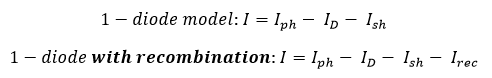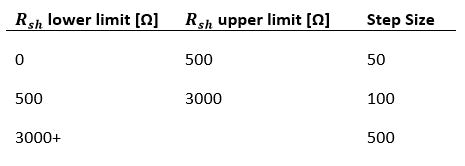# Default Algorithm

This algorithm is used to generate a module file using basic datasheet inputs and default assumptions. At its core is the single diode equivalent circuit model for photovoltaic modules (as defined by the circuit diagrams below for modules with and without recombination; the recombination current path is shown as a dotted line).The equation governing this equivalent circuit is achieved using Kirchoff’s current law, which states that current must sum to zero at any junction of a circuit:The resulting governing equations are:and all other variables are defined below in “Inputs”. The particular application of the single diode model is based on a process and set of assumptions developed by Andre Mermoud. All of the required inputs can be sourced from a standard module datasheet from any manufacturer.1. Calculate shunt resistance and dark shunt resistance.

The default shunt resistance is calculated according to the following equation quantized (rounded) to a step size dependent on what range the raw calculated value lies in.…where K is the “shunt resistance multiplier”, a technology specific multiplier (defaulted to 5.0 for c-Si modules and 3.0 for CdTe modules). The table below specifies the quantization (rounding interval) for each range of raw shunt resistance values.Rsh,dark resistance is calculated by multiplying Rsh by a constant and quantized similarly to shunt resistance.where Kdark is the dark shunt multiplier. The table below specifies the quantization (rounding interval) for each range of raw  values.2. Calculate the recombination parameter if the Model Type is “1-Diode with Recombination” (skip otherwise).

If generating a module with recombination, the recombination parameter must be estimated, which is derived from the maximum recombination parameter. In order to find the maximum recombination parameter, series resistance is set to (approximately) zero. The algorithm then increments recombination parameter, starting at zero, and solves a system of equations at each iteration.

The system of equations is defined using the “1-diode with recombination” governing equation above at 3 different conditions: (I = Isc, V = 0), (I = 0, V = Voc), and (I = Imp, V = Vmp). The Levenberg-Marquadt non-linear least squares solving algorithm is used to solve the system of equations and calculate the following unknowns: Iph (light generated current), γ, and I0. The initial guesses for solving the system of equations are as follows:The iterative loop ends when the condition I0/Iph < 1 x 10-12 is met. The value of recombination parameter (di2/μτ) from the previous iteration before this limit is reached is taken to be the maximum recombination parameter. The final value for the recombination parameter is calculated using the simple relationship:3. Calculate maximum series resistance.

The next step is to calculate the maximum theoretical series resistance to serve as an upper limit for calculating the actual series resistance. The algorithm iterates through incrementally increasing values of Rs, starting from (nearly) zero. Upon each iteration, a system of equations is solved to determine the optimal values for unknowns, similarly to step 2. The appropriate governing equation (“1-diode model” or “1-diode with recombination”) should be used. Additionally, for the non-recombination “1-diode model” use case, the only unknowns calculated from the system of equations are I0 and γ. Light-generated current is calculated with the following equation:where Rs,i is the ith iteration’s series resistance value. The iterative loop ends when either no solution can be found, or the ratio I0/Iph < 1 x 10-12. This ratio is a somewhat arbitrarily, but empirically-determined limit. The value of Rs in the previous iteration before this limit is reached is Rs,max.

4. Calculate series resistance and final single diode parameters.

1-diode model (no recombination)

For the “1-diode model” use case, determine the series resistance value that will yield 97% relative efficiency at 200 W/m2 and 25⁰C. The algorithm does this by iterating through values of Rs between 20% and 95% of Rs.max (determined in the previous step). Upon each iteration, the system of equations is solved similarly to previous steps using the “1-diode model” governing equation and the same three conditions. The solution to the system of equations is used to calculate of the linear dependence of γ (µγ) (see separate documentation for the µγ calculation). The relative efficiency at 200 W/m2 is then calculated (see separate documentation for the EIR algorithm).

At the end of each iteration, error between the calculated EIR value at 200 W/m2 and the desired value of 0.97 is recorded. The error calculated in the current iteration is compared to the previous iteration. If the sign of the error changes (i.e. positive to negative error), then the value of Rs from the previous iteration is chosen as optimal.

The values for I0, γ, and μγ calculated in the iteration corresponding to the optimal Rs value are the final single diode parameters.

1-diode Model with Recombination

In the case of recombination, series resistance is calculated using the simple relationship:The values for I0 and γ by solving the appropriate system of equations with the final value of series resistance, and used as inputs for calculating μγ.